×

Search anything:

# Monotone Chain algorithm for Convex Hull

#### computational geometry AlgorithmsGet this book -> Problems on Array: For Interviews and Competitive Programming

In this article, we have explored Monotone Chain algorithm for finding Convex Hull in a given set of points.

• Introduction
• Approach
• Algorithm
• Time Compexity
• Applications
• Overview

# Introduction

In this article, we shall cover the monotone chain algorithm for finding the convex hull for a set of points.

The convex hull of a set of points is the smallest convex polygon that can contain all the points in a given space. The monotone chain algorithm is an effective method for this, based on splitting the shape into an upper and lower hull.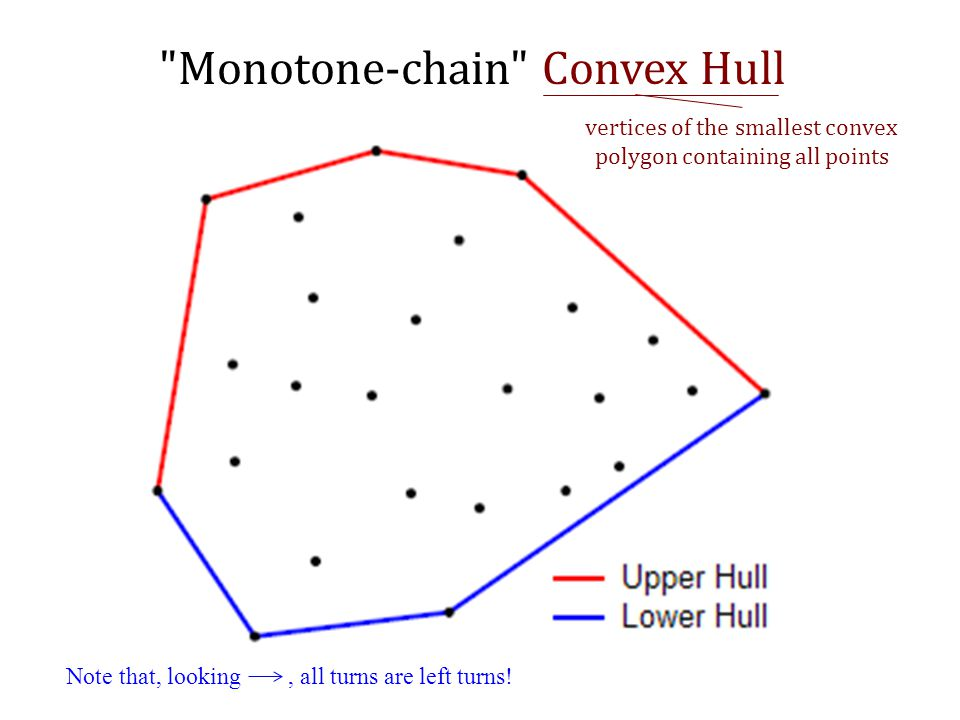# Approach

The monotone chain algorithm works in the following steps:

• Sort the points with respect to their x-coordinates (y if there is a tie in the x plane).
• The upper and lower hulls are then calculated in O(n) time.
• Find the leftmost point, rotate clockwise to find the next point and repeat until the right most point is found. Rotate again clockwise to find the lower hull.

In pseudocode, this can be seen below:

``````Input: list of points P

Sort the points of P by x-coordinate (in case of a tie, sort by y-coordinate)

Initialize U and L as empty lists. These will hold upper and lower hulls

for i = 1, 2, ..., n:
while L contains at least two points and the sequence of last two points
of L and the point P[i] does not make a counter-clockwise turn:
remove the last point from L
append P[i] to L

for i = n, n-1, ..., 1:
while U contains at least two points and the sequence of last two points
of U and the point P[i] does not make a counter-clockwise turn:
remove the last point from U
append P[i] to U

Remove the last point of each list (since it is identical to the first)
Concatenate L and U to obtain the convex hull of P.
``````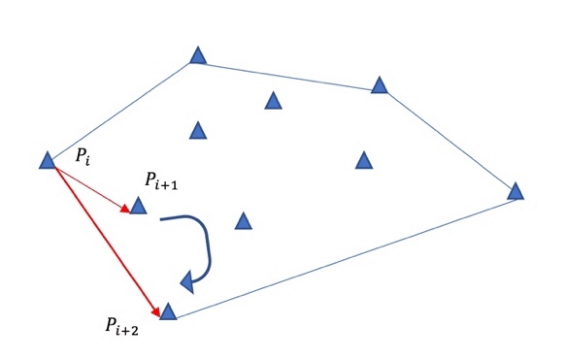# Algorithm

We shall now implement this into Python.

``````def convex_hull(points):
# Sort the points lexicographically (tuples are compared lexicographically).
# Remove duplicates to detect the case we have just one unique point.
points = sorted(set(points))

# In the case of 1 or no points
if len(points) <= 1:
return points

# Computes 2D cross product of vectors OA and OB
# Return positive value for OAB -> anti-clockwise turn
# Return negative value for OAB -> clockwise turn
# Return 0 for OAB -> points are colinear
def cross(o, a, b):
return (a - o) * (b - o) - (a - o) * (b - o)

# Construct lower hull
lower = []
for p in points:
while len(lower) >= 2 and cross(lower[-2], lower[-1], p) <= 0:
lower.pop()
lower.append(p)

# Construct upper hull
upper = []
for p in reversed(points):
while len(upper) >= 2 and cross(upper[-2], upper[-1], p) <= 0:
upper.pop()
upper.append(p)

# Concatenate upper and lower hull to get convex hull
# Remove the last point from each last as it is repeated at the beginning
return lower[:-1] + upper[:-1]
``````

Firstly, we sort the input points lexicographically, and add an edge case for when 1 or no points are inputted. Then to decide which way to turn we find the cross product of vectors between two points, if it is positive, turn anti-clockwise and negative, clockwise. If this value is 0 then we can state the points are colinear. Next, we shall define and calculate the upper and lower hulls of the points (halves of the convex hull). And then concatenate them both in order to return the final convex hull.

For example, the convex hull of a 10x10 grid would be (0,0), (9,0), (9,9), (0,9).

The convex hull of [(0,3), (2,2), (1,1), (2,1), (3,0), (0,0), (3,3)] would be (0,0), (3,0), (3,3), (0,3).

# Time Complexity

The monotone chain algorithm for constructing the convex hull is completed in O(nlogn) time, The upper and lower hulls are calculated in O(n) time.

# Applications

Convex hulls (by extension monotone chain algorithm) has uses in many different fields, a number are listed below.

## Mathematics

A large use case for convex hulls is in maths. For example in the field of spectral analysis, the numerical range of a normal matrix is the convex hull of its eigenvalues. It can also be used in rendering of and calculations involving hyperbolic spaces. For example they can be used in the calculation of hyperbolic manifolds and low-dimensional topology. Another common use for convex hulls is the construction of Bezier curves, where it is known to lie within the convex hull of its control points.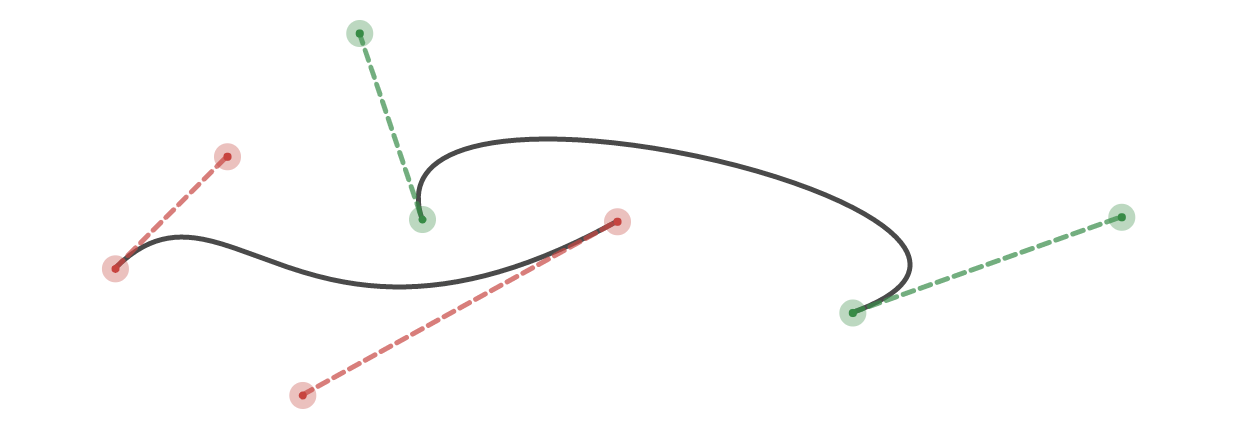## Quantum Physics

In quantum physics, the state-space (set of all possible configurations for a system) is a convex hull with extreme points as positive semi-definite operators with interior points as mixed states (pure and mixed states respectively). The Schrodinger-HJW theorem states that any mixed state can be written as a convex combination of pure states in multiple ways.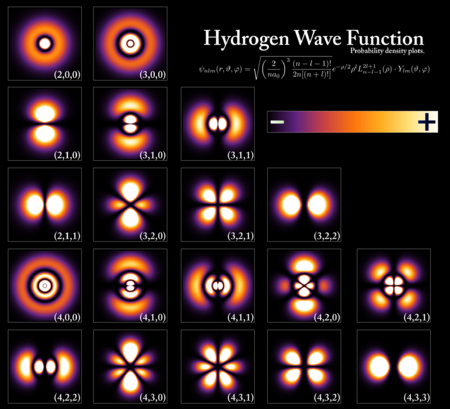## Computer Vision

Convex hulls are extensively used in computer vision for calculating the approximate space/area that an object takes up. This is mostly useful in applications such as self-driving cars eg. if a convex hull is calculated of surrounding objects and the vehicle itself, an algorithm can then check for any intersections between the hulls to avoid collisions. This is much easier for computers to calculate as opposed to if much denser point amounts were used for each object. By extension this can also therefore be applicable in robot motion planning and additionally pose tracking such as seen in the image below.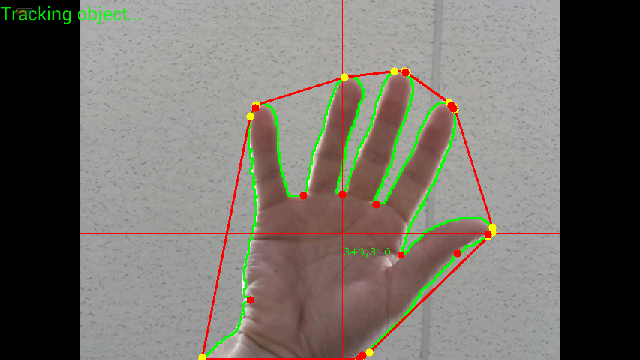# Overview

In this article at OpenGenus, we have discussed the monotone chain algorithm for finding the convex hull of a set of points. We explained the basis of a convex hull and the algorithm itself, layed out each step, implemented it in Python and explained its performance before finally talk through some of the many use cases for a convex hull in the real world.